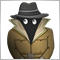# Need help! (Basic code)1116

Hi experts,

I have learnt about mql4 and stuck with "for" loop.plz see the script.

```void OnStart()
{

int e =2;
for(int i=1;i<3;i++)
{
for(int j=i;j<j+e;j++)
{
Print(j);
}
}

}```

It's not complex at all.But The result maked me so confuse.

I think the shown value should be 1,2,2,3. are they correct?

Thank you.7863

```int e =2;
for(int j=i;j<j+e;j++)```

This is an endless loop. You get TRUE all the time.1116

Konstantin Nikitin:

This is an endless loop. You get TRUE all the time.

Thank you Konstantin